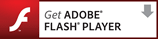## Statistics

You need Adobe Flash Player to view some content on this site.Analysis of data can be simple or complex as you decide what tools to use to apply to the data. You can simply count, sort, and order the pieces of data. You can perform statistical tests to determine the relationship between two sets of data, or use the information to try to find patterns that allow you to predict future behavior. Whichever tools you use for analysis, be sure to continue to ask yourself, “to what end?” and make sure that the analysis is used in service of the mission of your program.

### It is useful to be familiar with common descriptive statistics.

It is likely that most analysis will use measures of total, arithmetic mean, and standard deviation. However, it is useful to be familiar with all the descriptive statistics.

Measures

Total - the total is the sum of all parts.

Considerations: The total by itself is less useful than if used by comparison to show increases. For example, the total number of organizations served, the total number of youth participating in a program, or the total amount of additional funds raised by an organization.

Arithmetic Mean - The arithmetic mean is the sum of the observations divided by the number of observations. It is the most common statistic of central tendency, and when someone says simply "the mean" or "the average," this is what they mean.

Considerations: This measurement is easily skewed by outrageous outliers. For example, if you are reporting the average additional funds that organizations raised, if one organization was able to raise many times the amount of others, the average goes up significantly.

Median - The median is found by sorting all the data from lowest to highest, and taking the value of the number in the middle. If there is an even number of observations, the median is the average of the two numbers in the middle.

Considerations: If the distribution of data is very skewed, the median is a more useful tool to indicate the central tendency because it is less influenced by outliers.

Mode - The mode is the common value in the data set.

Considerations: Mode is particularly useful when you have data that is grouped into a small number of classes, for example, the type of organization you are serving, or what county the organization operates in. The mode is simply the type of organization you serve most frequently, or the county where the largest number of organizations operate.

Standard Deviation - Standard deviation is a measure of the variability or dispersion of a data set.

Considerations: A low standard deviation indicates that the data points tend to be very close to the same value (the mean), while high standard deviation indicates that the data are spread out over a large range of values.  For example, if looking at the amount of money each organization devoted to hiring consultants, if the range of dollars spent was between \$40-\$200 dollars, the standard deviation would be low compared to a range of \$20 - \$300,000.

Ratio - A ratio is an expression that compares quantities relative to each other.

Considerations: A ratio is a proportional relationship and therefore compares two variables against each other.

### Test your knowledge of descriptive statistics.

You need Adobe Flash Player to view some content on this site.### Inferential statistics help you draw conclusions that may be more widely applicable.

Where descriptive statistics help you understand the data as you have it, inferential statistics help you draw conclusions that may be more widely applicable beyond the specific data set you are working with. Essentially, inferential statistics allow you to “infer” additional information. Two common operations are correlation and regression.

Correlation uses statistical formulas to calculate the relationship between two variables. For example, the two variables might be the number of hours of technical assistance an organization receives and the capacity index score at the beginning or end of the intervention, or even the difference between the two. You might expect that organizations with lower capacity index scores required more technical assistance, or that those who received more hours of technical assistance saw more increases in capacity index scores. The statistical methods of calculating correlative relationships help define the degree of interdependence between the two variables. Regression is the process of plotting the two variables on a graph, and then finding a line that “best fits” the trends in the data. It helps predict what values you might expect to see in one variable given another variable.

For both correlation and regression, it is important to understand that the equations do not definitively provide evidence of a cause and effect relationship. For example, number of hours of technical assistance may go up as the capacity index score decreases (a negative relationship). One possible explanation would be that your capacity building program design requires that you spend more time with organizations with lower capacity scores. On the other hand, more hours of technical assistance may be associated with organizations with lower capacity scores because those organizations did not exhibit high levels of readiness for change.